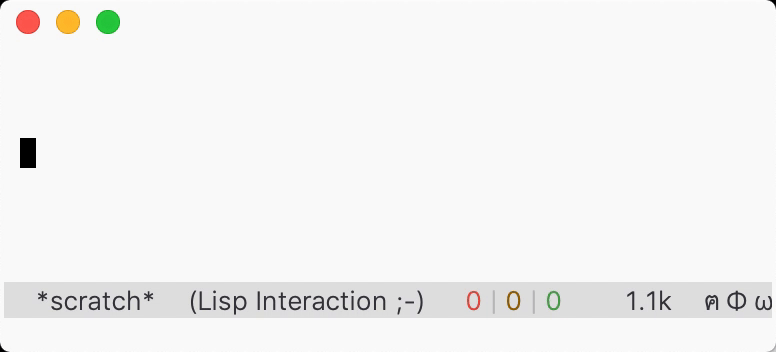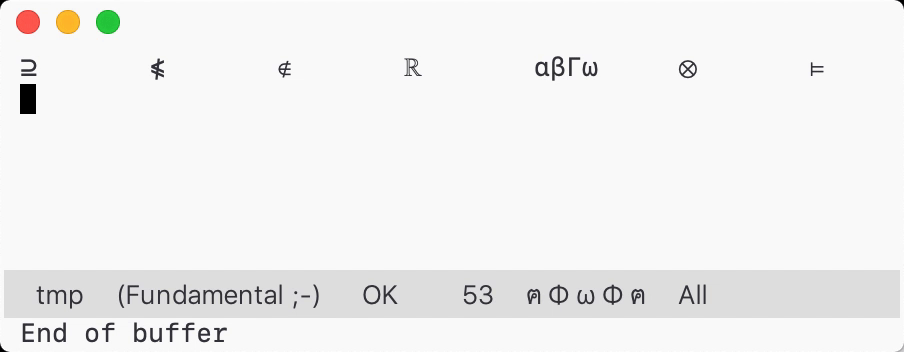# 类似TeXmacs转化特殊字符的命令``````(defvar transform-list (list (mapcar #'char-to-string "*×·⊗⊙")
(mapcar #'char-to-string "*123456"))
"Each car is a starting char, cdr is a list of transformations.")

(defun transform-get-variant-list (char)
"Find CHAR in ‘transform-list’, return (index . variant-list).
Return nil if none found. CHAR should be a string."
(catch 'ret
(dolist (variant-list transform-list nil)
(cl-loop for variant in variant-list
for idx from 0 to (1- (length variant-list))
if (equal variant char)
do (throw 'ret (cons idx variant-list))))))

(defun transform--make-step-fn (variant-list init-idx)
"Return a stepping function that steps through each variation.
At first the index is INIT-IDX.
VARIANT-LIST is a list of variant characters.

The step function takes a integer “step” that changes the index
of current variant, e.g. 1 is next, -1 is prev. It returns the
current index after adding the “step” with current index."
(let ((variant-index (or init-idx 0)))
(lambda (step)
(setq variant-index (+ step variant-index))
(when (eq variant-index (length variant-list))
(setq variant-index 0))
(when (< variant-index 0)
(setq variant-index (1- (length variant-list))))
(atomic-change-group
(delete-char -1)
(insert (nth variant-index variant-list)))
(message "%s" (transform-make-message variant-list
variant-index))
variant-index)))

(defun transform-make-message (variant-list index)
"Make a string that displays each variant in VARIANT-LIST.
Highlight the one marked by INDEX."
(string-join (cl-loop for variant in variant-list
for idx from 0 to (1- (length variant-list))
if (eq idx index)
collect (propertize variant 'face 'highlight)
else collect variant)
" "))

(defun transform-previous-char ()
"Transform char before point."
(interactive)
(when-let ((c (transform-get-variant-list (char-to-string
(char-before)))))
(let* ((index (car c))
(variant-list (cdr c))
(step-fn (transform--make-step-fn variant-list index))
(map (let ((map (make-sparse-keymap)))
(define-key map (kbd "C-n")
(lambda () (interactive) (funcall step-fn 1)))
(define-key map (kbd "C-p")
(lambda () (interactive) (funcall step-fn -1)))
(define-key map (this-command-keys)
(lambda () (interactive) (funcall step-fn 1)))
map)))
(funcall step-fn 1)
(set-transient-map map t))))
``````
7 个赞

``````(defun toki/strip-modifier (key)
"Strip the modifier of KEY, and return the result.
KEY is an integer representing the key event, and so does the
return value.

Some keys are considered to have a modifier by
Emacs, like \"RET\" is the same as \"C-m\". Such keys are not
modified by this function."
(if (member (key-description (make-vector 1 key))
'("RET"))
key
(event-convert-list
(append (cl-set-difference
(event-modifiers key)
'(control meta shift))
(list (event-basic-type key))))))
``````

``````(setq keys (this-command-keys-vector))
=> [24 5]
(setq key-event
(make-vector 1
(toki/strip-modifier
(aref keys (1- (length keys))))))
=> 
(message (format
"Press %s to run command again"
(key-description key-event)))
=> 显示 "Press e to run command again"
``````

1 个赞

``````(message (char-to-string
(event-basic-type
(car (last (listify-key-sequence
(this-command-keys)))))))
``````1 个赞``````(defvar transform-list
(mapcar (lambda (x) (mapcar #'char-to-string x))
(split-string (string-join
'("*×·⊗⊙ +⊕ |⊦⊨ /÷ \\∖"
"<∈⊂⊏ >∋⊃⊐ =≈"
"v∨ ^∧ 0∅"
"Rℝ Zℤ Qℚ Nℕ Cℂ"
"aαΑ∀ bβΒ gγΓ dδΔ eεΕ∃ zζΖ hηΗ qθΘ"
"iιΙ kκΚ lλΛ mμΜ nνΝ∩ xξΞ oοΟ pπΠ"
"rρΡ sσΣ tτΤ yυΥ fφΦ cχΧ uψΨ∪ wωΩ")
" ")))
"Each element of the list is a list of related variants.")

(defun transform-get-variant-list (char)
"Find CHAR in ‘transform-list’, return (index . variant-list).
Return nil if none found. CHAR should be a string."
(catch 'ret
(dolist (variant-list transform-list nil)
(cl-loop for variant in variant-list
for idx from 0 to (1- (length variant-list))
if (equal variant char)
do (throw 'ret (cons idx variant-list))))))

(defun transform--make-step-fn (variant-list init-idx)
"Return a stepping function that steps through each variation.
At first the index is INIT-IDX.
VARIANT-LIST is a list of variant characters.

The step function takes a integer “step” that changes the index
of current variant, e.g. 1 is next, -1 is prev. It returns the
current index after adding the “step” with current index.

The step function with step across the variant list and change
the character before point to the current variant."
(let ((variant-index (or init-idx 0)))
(lambda (step)
(setq variant-index (+ step variant-index))
(when (eq variant-index (length variant-list))
(setq variant-index 0))
(when (< variant-index 0)
(setq variant-index (1- (length variant-list))))
(atomic-change-group
(delete-char -1)
(insert (nth variant-index variant-list)))
(message "%s" (transform-make-message variant-list
variant-index))
variant-index)))

(defun transform-make-message (variant-list index)
"Make a string that displays each variant in VARIANT-LIST.
Highlight the one marked by INDEX."
(string-join (cl-loop for variant in variant-list
for idx from 0 to (1- (length variant-list))
if (eq idx index)
collect (propertize variant 'face 'highlight)
else collect variant)
" "))

(defun transform-previous-char ()
"Transform char before point.

If previous char is “/” or “_”, apply ‘accent-previous-char’
(interactive)
(if (member (char-before) '(?/ ?_))
(accent-previous-char)
(if-let ((c (transform-get-variant-list (char-to-string
(char-before)))))
(let* ((index (car c))
(variant-list (cdr c))
(step-fn (transform--make-step-fn variant-list index))
(map (let ((map (make-sparse-keymap)))
(define-key map (kbd "C-n")
(lambda () (interactive) (funcall step-fn 1)))
(define-key map (kbd "C-p")
(lambda () (interactive) (funcall step-fn -1)))
(define-key map (this-command-keys)
(lambda () (interactive) (funcall step-fn 1)))
map)))
(funcall step-fn 1)
(set-transient-map map t))
(user-error "No possible transformation"))))

(defvar accent-list
'((?_ . "<≤ ⊂⊆ ⊏⊑ >≥ ⊃⊇ ⊐⊒")
(?/ . "=≠ <≮ ≤≰ ∈∉ ⊂⊄ ⊆⊈ >≯ ≥≱ ∋∌ ⊃⊅ ⊇⊉")))

(defun accent-previous-char ()
"Accent previous char by its trailing accent modifier."
(interactive)
(let ((modifier-list (mapcar #'car accent-list)))
(if (not (member (char-before) modifier-list))
;; base case, prev char is normal char
nil
;; recursion case  <char><mod>|
(let ((modifier (char-before))
old-char new-char)
(atomic-change-group
(delete-char -1)
(accent-previous-char)
(setq old-char (char-before))
;; find accented char
(setq new-char (condition-case nil
(with-temp-buffer
(insert (alist-get modifier accent-list))
(goto-char (point-min))
(search-forward (char-to-string old-char))
(char-after))
(search-failed nil)))
(if (not new-char)
(user-error "Accent doesn’t exist")
(delete-char -1)
(insert new-char)))))))
``````

2 个赞

1 个赞